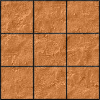#### You may also like### Pyramids

What are the missing numbers in the pyramids?### Paving the Way

A man paved a square courtyard and then decided that it was too small. He took up the tiles, bought 100 more and used them to pave another square courtyard. How many tiles did he use altogether?### Chess

What would be the smallest number of moves needed to move a Knight from a chess set from one corner to the opposite corner of a 99 by 99 square board?

# The Simple Life

##### Age 11 to 14 Challenge Level:

Lily from Jersey College for Girls found another possibility:
I found one possibilty for this question, and my answer became $3(x+2y) + 2(x+y)$, this would lead to $3x+6y + 2x+2y$, then the answer becoming $5x + 8y$.

Minjoon from Seoul Foreign British School found another one:
$a(x+y)+b(x-2y)$

$6x+6y-x+2y=5x+8y$
(so $a=6$ and $b=-1$)

Brian found a possibility using Alison's algebraic method:
$$a(x+2y)+b(2x+3y)=5x+8y\\\Rightarrow\begin{cases}ax+2bx=5x \\2ay+3by=8y\end{cases}\\ \Rightarrow\begin{cases} a+2b=5\\2a+3b=8\end{cases}\\ \Rightarrow a=1\hspace{4mm}\text{and}\hspace{4mm}b=2$$

Rishika from Nonsuch High School for Girls used her own algebraic method, similar to Alison's, to find all of the possibilities:
I used an algebraic method to find the multiples using the following method:
For example, $$a(x+y)+b(x+2y) = 5x+8y$$
{$a$ and $b$ represent the values of the multiples we need to find}

We can then form two equations if we substitute $x=0$ and $y=0$,
When $x=0$,
$$ay+2by = 8y$$
When $y=0$,
$$ax+bx = 5x$$
Then, we can cancel the like terms in each equation:

Equation 1: $a+2b = 8$

Equation 2: $a+b = 5$

Which we can solve simultaneously:

Equation 1 - Equation 2 (as '$a$' is present in both): $b = 3$

Substitute into Equation 2: $a = 2$

Therefore, $2 (x+y) +3 (x+2y) = 5x+8y$

I repeated this for all other possibilities.
Rishika made some mistakes, but these are the ones that Rishika found correctly:
\begin{align}2 (x+y) &+3 (x+2y) \hspace{4mm}\text{{from above}}\\ 6 (x+y) &-1 (x-2y)\\ 4 (x+y) &+1 (x+4y)\\ 1 (x+2y) &+2 (2x+3y)\\ \end{align}

Rishika identified all of the possible pairs of expressions. Can you use Rikisha's method, or any other method, to find multiples of these pairs that add up to $5x+8y$?
$(x+y)$ and $(2x+3y)$
$(x+2y)$ and $(x-2y)$
$(x+2y)$ and $(x+4y)$
$(x-2y)$ and $(x+4y)$
$(x-2y)$ and $(2x+3y)$
$(x+4y)$ and $(2x+3y)$